Author
Taylor HartleyExpert Reviewer

Published: August 24, 2023Author
Taylor HartleyExpert Reviewer

Published: August 24, 2023Author
Taylor HartleyExpert Reviewer

Published: August 24, 2023Key takeaways

• Its properties are important – There are certain criteria a shape must meet to be considered a quadrilateral.
• There are different types – A quadrilateral isn’t its own shape, but rather a family of shapes including squares, rectangles, and kites that all share the same properties.
• They can be regular or irregular, and convex or concave – In fact, the only shape that is a perfectly regular quadrilateral is the square!A quadrilateral is a polygon that has four sides, four angles, and four vertices. An easy way to remember this is to think of the word “quad” in quadrilateral which means “four.” If you’re having trouble remembering what “quad” stands for, just think of a motorized quad that has four tires!

Here is an example of a quadrilateral: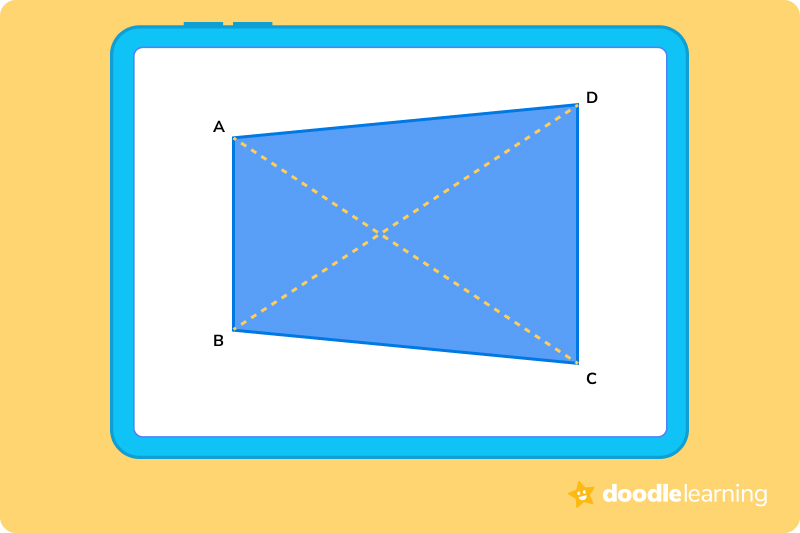Check out each part of a quadrilateral, and reference the shape above to visualize the components:

• ∠A, ∠B, ∠C, and ∠D are the four angles of the quadrilateral ABCD
• AB, BC, CD, and DA are the four sides of the quadrilateral ABCD
• A, B, C, and D are the four vertices of the quadrilateral ABCD
• AC and BD are the two diagonals of the quadrilateral ABCDNow that you know what a quadrilateral is and what its properties are, let’s take a look at some real-world examples: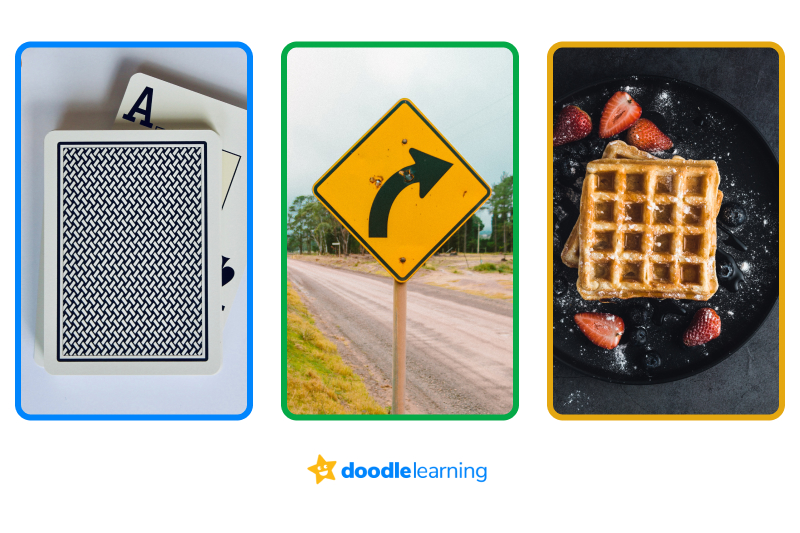Keep in mind, any shape that does not have four sides or four angles, or has open sides is not a quadrilateral.

## Must-have properties of a quadrilateralBefore we dive into the different types of quadrilaterals, let’s go over a quick list of the common quadrilateral properties — these will be important to remember throughout the practice problems below!

• Has four vertices and angles
• Has four sides
• The sum of all interior angles is 360°
• Has two diagonals
• Can be regular or irregular. A regular quadrilateral has to have 4 equal sides and 4 equal angles, and its diagonals must bisect each other — a square meets these conditions, therefore it’s a regular quadrilateral.The quadrilateral example that you saw above is only one example out of several. Remember, as long as a shape meets all of the qualifications, it can be a quadrilateral! Here are the different types of quadrilaterals — parallelograms, squares, rectangles, and rhombuses — with picture examples and properties for each.

### ParallelogramA parallelogram has opposite sides that are parallel and equal, meaning that its opposite sides are two lines in the same plane that are equal distance from each other and never meet and they measure the same length.  Its opposite angles are equal, too. Squares, rectangles, and rhombuses are also parallelograms.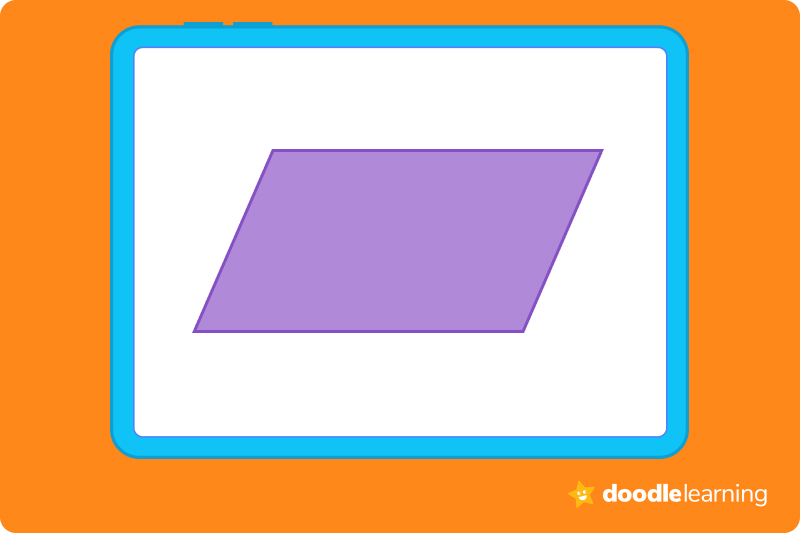### RectangleA rectangle has opposite sides that are parallel and equal, like the parallelogram. And all angles are equal and measure 90°.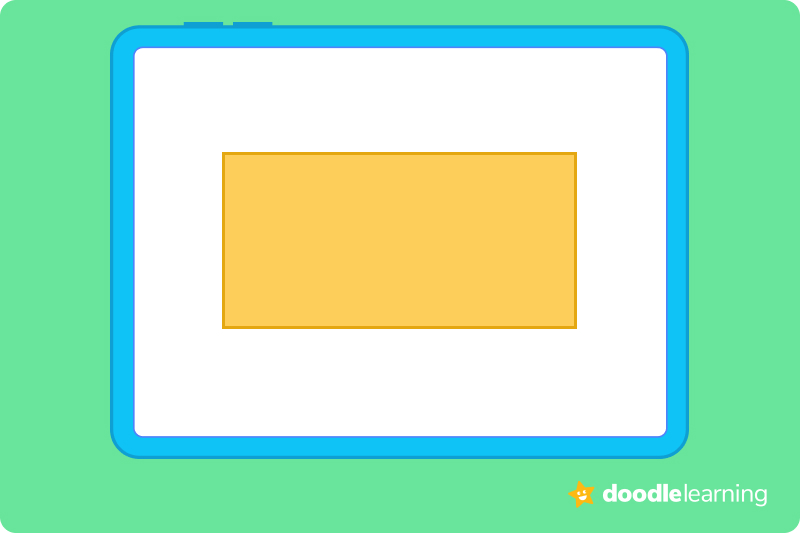### RhombusA rhombus is a shape that has all equal sides, and its opposite angles are equal. You might find that definition similar to a square, and it is! But, the difference between a square and a rhombus is that all angles of a square are right angles, but the angles of a rhombus do not have to be right angles. Therefore, every square is a rhombus, but all rhombuses are not squares.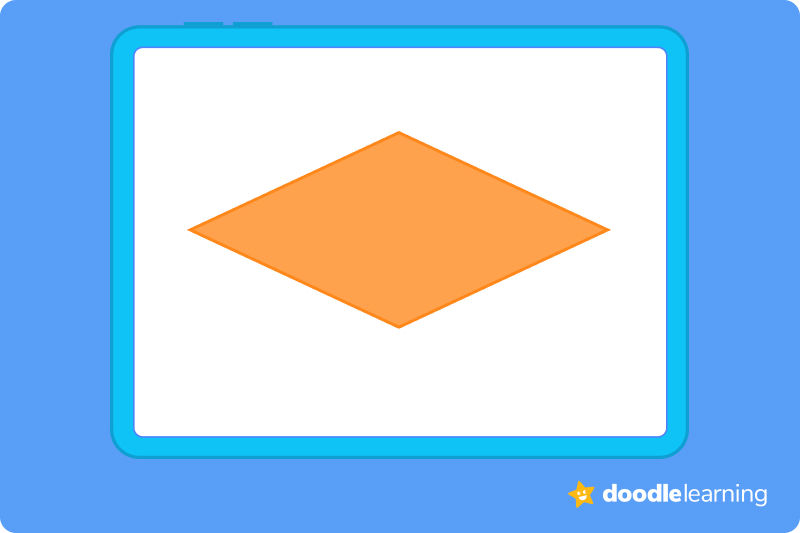### SquareA square is a shape that has all equal sides and angles, and all angles measure 90°.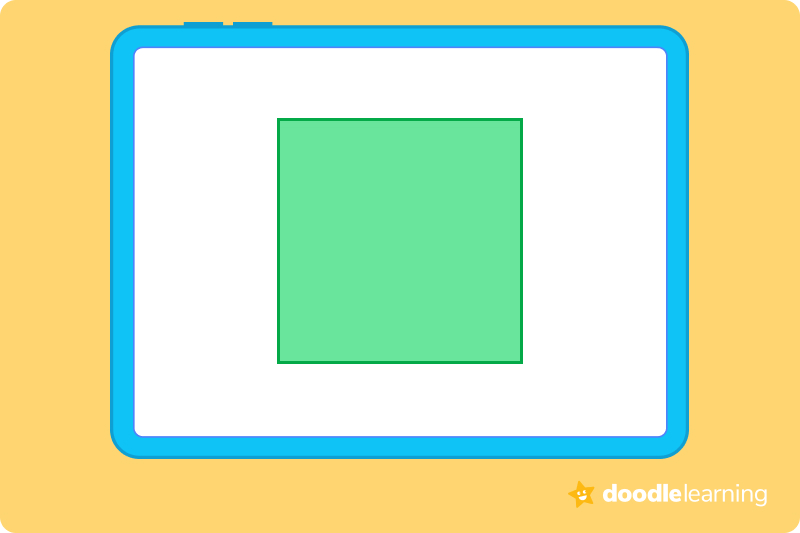### TrapezoidA trapezoid has one pair of opposite sides that are parallel, and its adjacent angles between the non-parallel sides add up to 180°.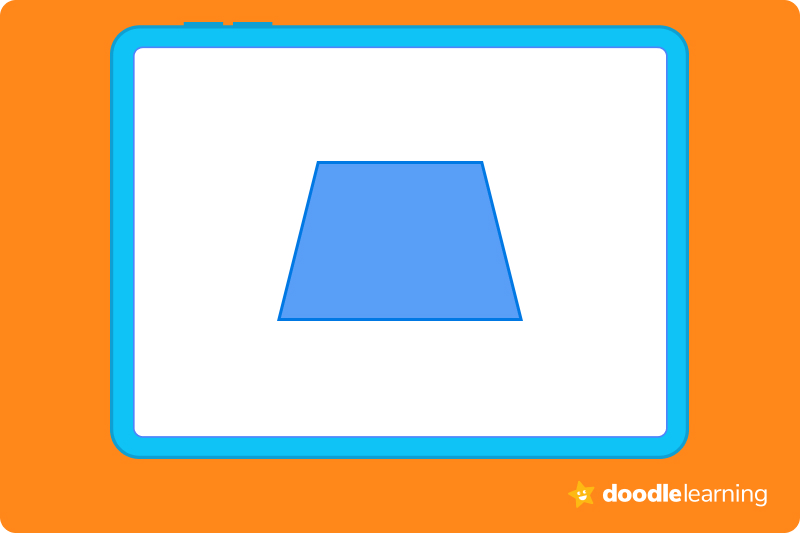### KiteA kite is a shape, too! It has equal adjacent sides, and one pair of opposite angles are equal.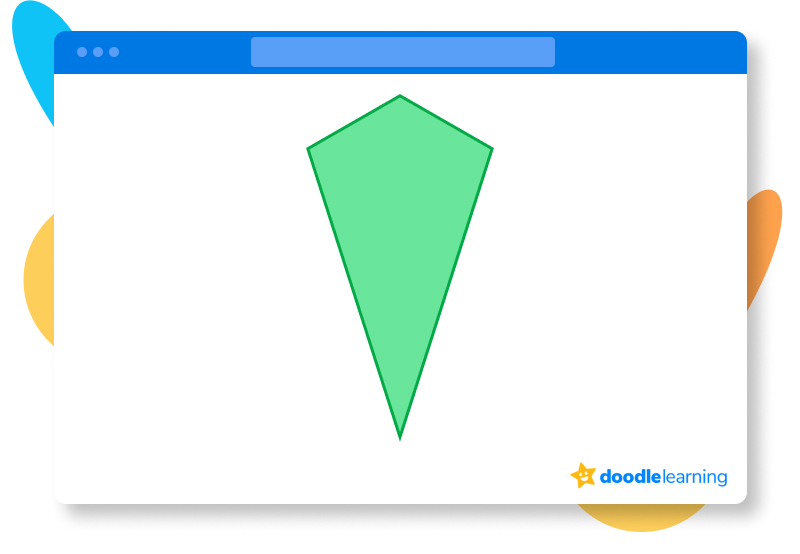Next, let’s establish the difference between convex and concave quadrilaterals.

• Concave quadrilaterals — One interior angle is greater than 180°.
• Convex quadrilaterals — Each interior angle is less than 180°. A quadrilateral is convex if its two diagonals both lie completely within the shape..

Here’s an example of each: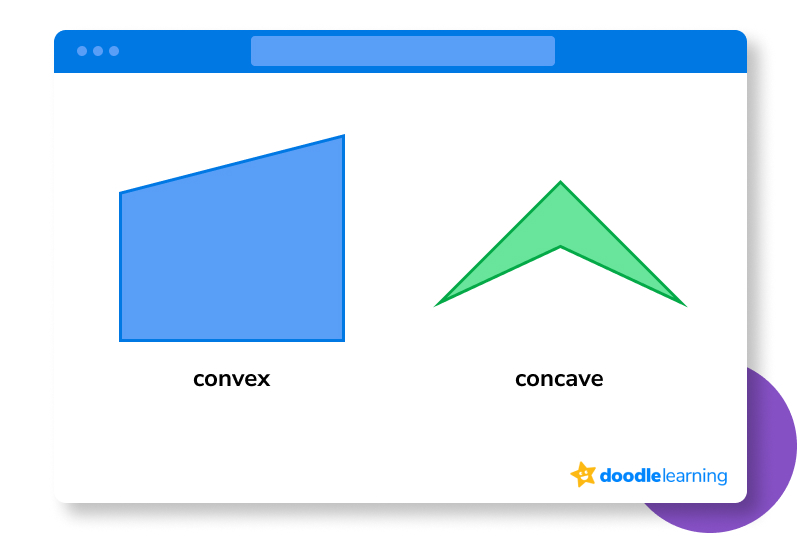Once you can recognize a quadrilateral and name its properties, you might be asked to find its perimeter and area — so let’s find out how!The perimeter of a quadrilateral is simply the length of its boundary. In other words, it’s the sum of all of its sides. If ABCD is a quadrilateral, then its perimeter can be expressed as ABCD = AB + BC + CD + DA.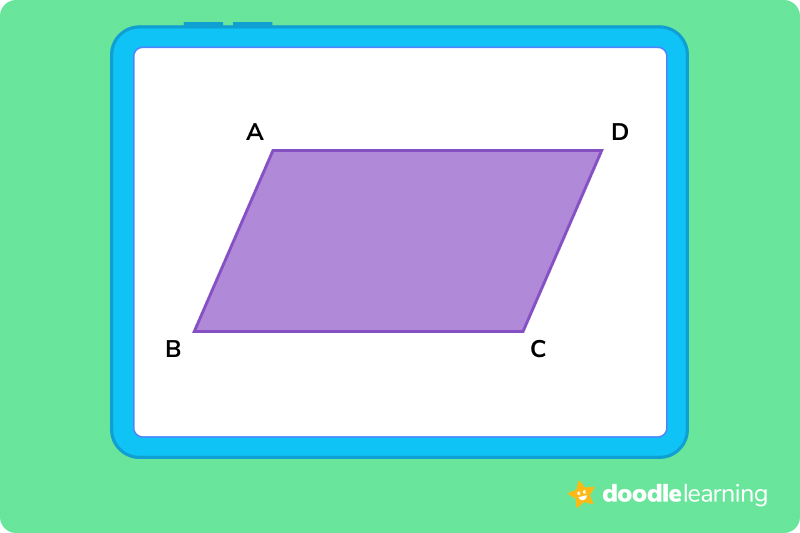Take a look at some of the different equations you can use to find the perimeter for various types of quadrilaterals:

• Rectangle — 2 x (length + width)
• Square — 4 x side
• Rhombus — 4 x side
• Parallelogram — 2 x sum of adjacent sides
• Kite — 2 x sum of adjacent sidesThe area of a quadrilateral is just the measurement of the region that’s enclosed by its sides. There are different formulas you have to use for each type of quadrilateral:

Rectangle — b x h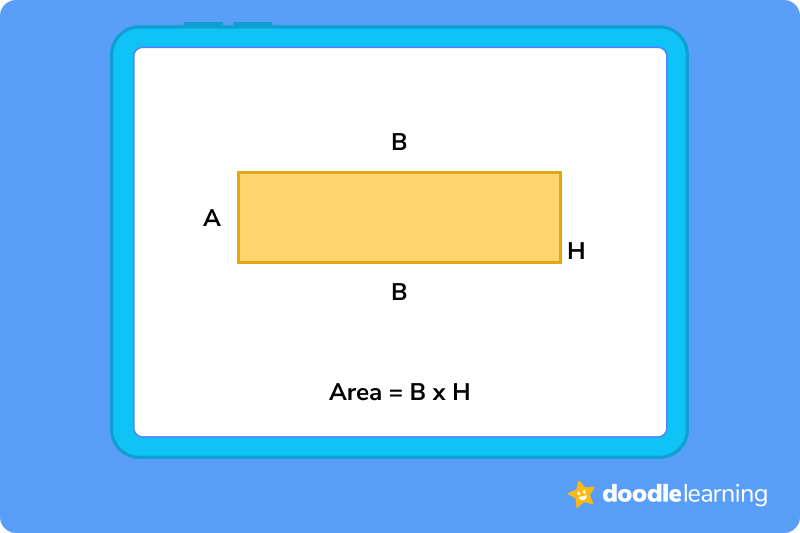Square — x2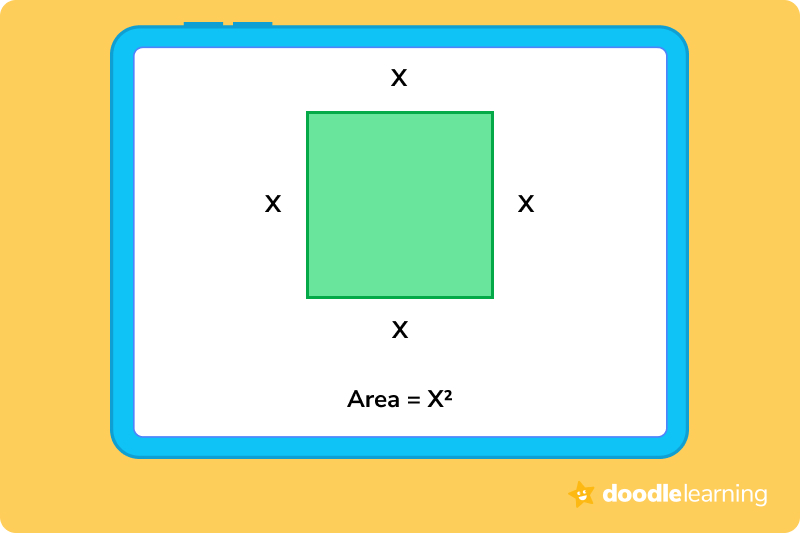Trapezoid — b x h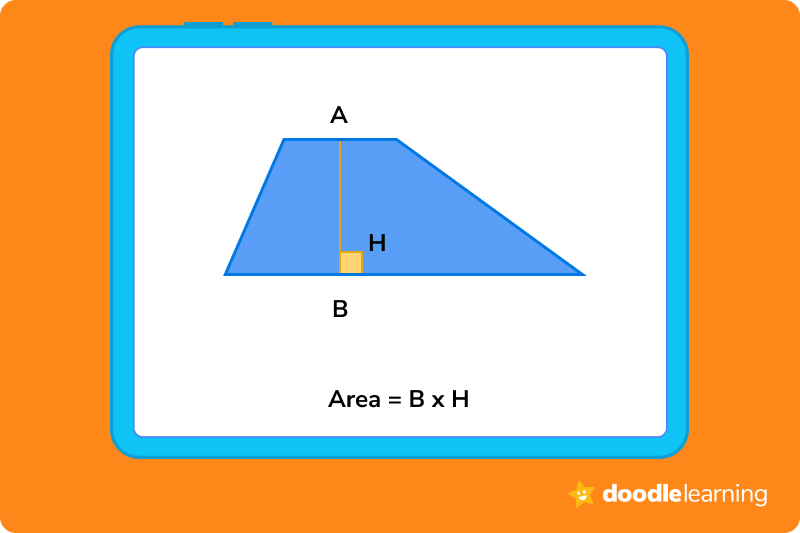Parallelogram — b x h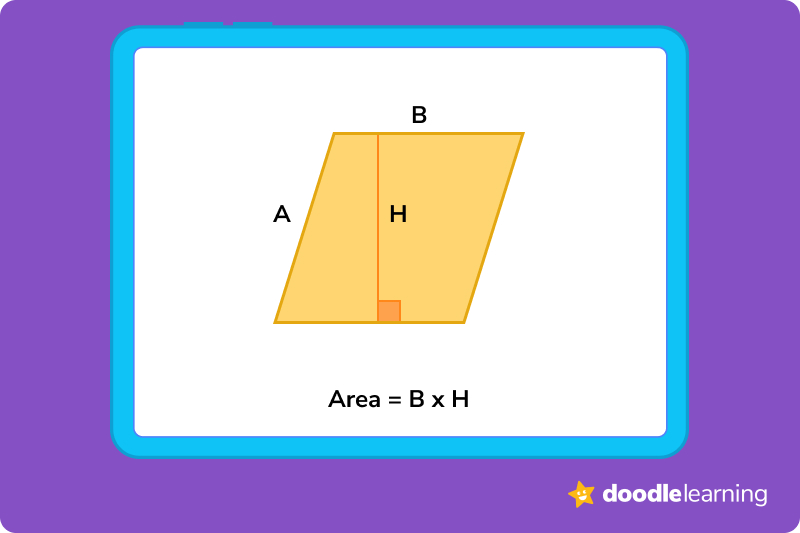Kite — ½ x d1 x d2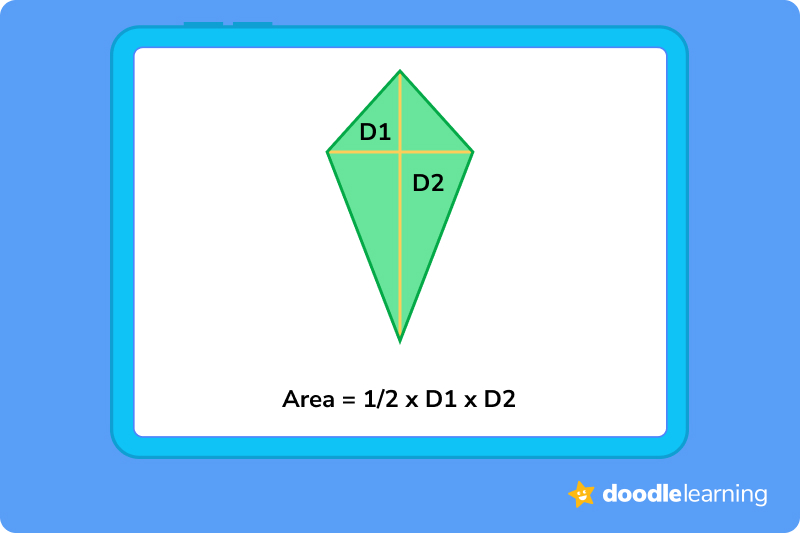Rhombus — ½ x (a + b) x h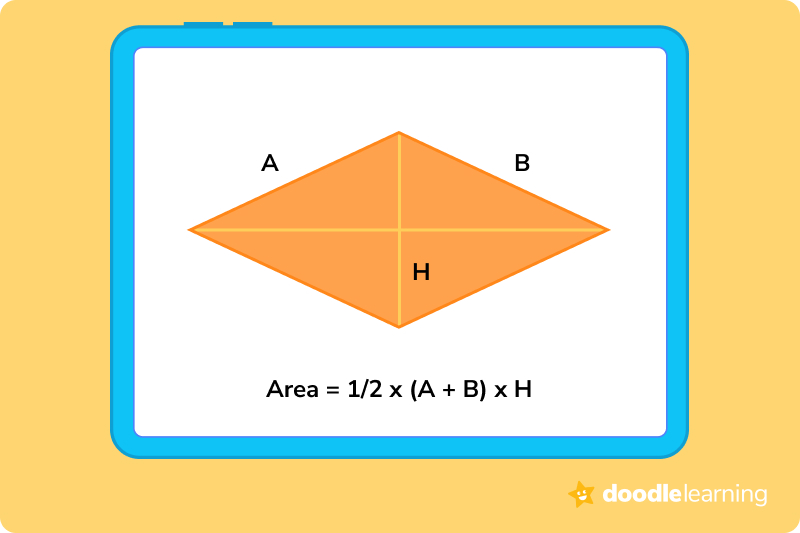## Let’s practice together!### 1. Find the missing angle ∠C in the following quadrilateral: ∠A = 72° ∠B = 53°, ∠D = 61°First, it might be helpful for you to draw a quadrilateral and label four angles with the measurements in the problem. One angle, ∠C, will equal x since that is the measurement we’re trying to find.

We know that all the angles of a quadrilateral must equal 360°. So we can add up all of the angles that we do know. 72 + 53 + 61 = 186.

Now, let’s subtract 186 from 360 to find the missing angle ∠C. 360 – 186 = 174.

∠C = 174°

### 2. What is the perimeter of a quadrilateral with the following side lengths: 10 cm, 6cm, 14cm, and 8cm?We know that the perimeter of a quadrilateral is just the sum of all of its sides. Therefore, we can add 10 + 6 + 14 + 8 to get 38. The perimeter of this quadrilateral is 38cm.

### 3. What is the area of a parallelogram with a base that equals 16 cm and a height that equals 12 cm?First, let’s find the equation for the area of a parallelogram which is b x h. We know that the base is 16 cm and the height is 12 cm, so we can rewrite the equation as 16 x 12 = 192. So, the area of this parallelogram is 192 cm2.

Ready to give it a go?

Now that we know the definition of a quadrilateral, the properties of a quadrilateral, and the different types of quadrilaterals, it’s time to put our new skills to the test! Work through the following problems on your own. Feel free to look back at the practice problems above if you get stuck or if you need a quick reminder on what a quadrilateral is.

And remember, don’t get discouraged if you run into a roadblock! Practice is the best way to learn something new.

## Practice problems

The perimeter is 32 cm.

## Parent Guide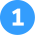It’s a rectangle

How did we get here?

We know that a rectangle is a type of quadrilateral, and its four angles all measure 90°. Plus, each and both the pair of opposite sides are equal. You could also say this could be a square, since it follows those same properties.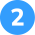The perimeter is 32 cm

How did we get here?

1. We know that the perimeter is simply the sum of all of a shape’s sides.
2. We also know that a square has 4 equal sides, and in this case, they’re each 8 cm.
3. We can write the equation for this problem as 4 x 8 or 8 + 8 + 8 + 8.
4. 4 x 8 = 32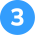True

How did we get here?

1. A quadrilateral can be regular or irregular.
2. A regular quadrilateral has to have 4 equal sides and 4 equal angles, and its diagonals must bisect each other — a square meets these conditions; therefore, it’s a regular quadrilateral.
3. An irregular quadrilateral has 4 sides and angles, but they do not all have to be equal. All quadrilaterals that are not squares fall into this category.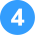84 cm2

How did we get here?

1. We know that to find the area of a rhombus using its base and height, all we have to do is multiply the base and height.
2. In this problem, we can write this as 12 x 7 = 84.
3. The area of this rhombus is 84 cm2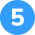8 units

How did we get here?

1. Here, we know that the area of the parallelogram is 48 square units and the height is 6 units.
2. We also know that the equation for the area of a parallelogram is base x height.
3. So, we have 48 = X x 6
4. To find the base, we can divide 48 by 6 which equals 8.
5. Now, we have the base as 8 units.
6. To check our work, simply multiply 8 units x 6 units to get 48 square units.

## FAQs about math strategies for kidsWe understand that diving into new information can sometimes be overwhelming, and questions often arise. That’s why we’ve meticulously crafted these FAQs, based on real questions from students and parents. We’ve got you covered!

There are six main types of quadrilaterals including parallelograms, squares, rectangles, rhombuses, trapezoids, and kites.

A quadrilateral has four vertices and sides, and the sum of all interior angles is 360°. They also have two diagonals.

No, all of the angles cannot be acute because then the sum of angles would be less than 360°.## Related Posts

Title 1

Title 2

Title 3

Lesson credits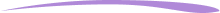Taylor Hartley

Taylor Hartley is an author and an English teacher based in Charlotte, North Carolina. When she's not writing, you can find her on the rowing machine or lost in a good novel.

Jill Padfield has 7 years of experience teaching high school mathematics, ranging from Alegra 1 to AP Calculas. She is currently working as a Business Analyst, working to improve services for Veterans while earning a masters degree in business administration.

Taylor Hartley

Taylor Hartley is an author and an English teacher based in Charlotte, North Carolina. When she's not writing, you can find her on the rowing machine or lost in a good novel.

Jill Padfield has 7 years of experience teaching high school mathematics, ranging from Alegra 1 to AP Calculas. She is currently working as a Business Analyst, working to improve services for Veterans while earning a masters degree in business administration.# Are you a parent, teacher or student?

Are you a parent or teacher?

## Hi there!

Book a chat with our team